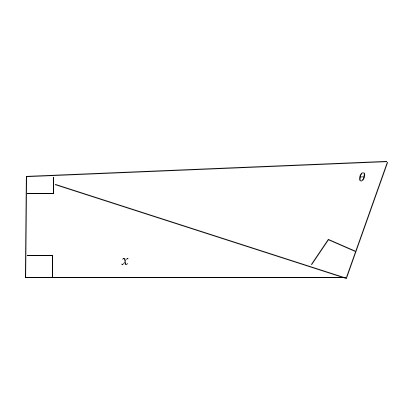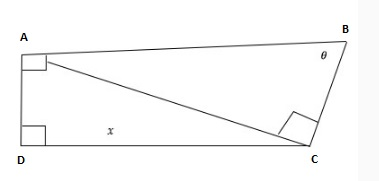# Express the length of x in terms of the trigonometric rations of \theta.

## Question:

Express the length of {eq}x {/eq} in terms of the trigonometric rations of {eq}\theta. {/eq}## Trigonometric Ratios :

With the help of the trigonometric ratios, we can find the length of the side of the triangle, quadrilateral, or any type of bounded quadrilateral or polygons.We just need to knwo that which angle is found for the trigonometric ratios.

We will first name the vertex of the quadrilateral as follows:Now using the tangent ratio we have:

Now when we have the length of AD known, then we can simply put it in the given expression of x,.